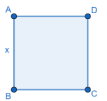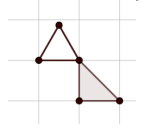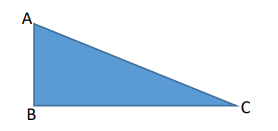# MA.912.T.1.2Export Print
Solve mathematical and real-world problems involving right triangles using trigonometric ratios and the Pythagorean Theorem.

### Clarifications

Clarification 1: Instruction includes procedural fluency with the relationships of side lengths in special right triangles having angle measures of 30°-60°-90° and 45°-45°-90°.
General Information
Subject Area: Mathematics (B.E.S.T.)
Strand: Trigonometry
Status: State Board Approved

## Benchmark Instructional Guide

### Terms from the K-12 Glossary

• Angle
• Equilateral Triangle
• Hypotenuse
• Isosceles Triangle
• Right Triangle

### Vertical Alignment

Previous Benchmarks

Next Benchmarks

### Purpose and Instructional Strategies

In grade 8, students solved problems involving right triangles using the Pythagorean Theorem. In Geometry, students use their understanding of sine, cosine and tangent to solve mathematical and real-world problems involving right triangles. In later courses, students will extend this knowledge to solve more difficult problems with right triangles, and extend the concept of trigonometric ratios to trigonometric functions on the unit circle and the number line.
• Within the Geometry course, the expectation is to use angle measures given in degrees and not in radians. Additionally, it is not the expectation for students to master the trigonometric ratios of secant, cosecant and cotangent within this course.
• It is customary to use Greek letters to represent angle measures (e.g., Ø, α, β, γ).
• Problem types include cases where some information about the side lengths or angle measures of a right triangle is missing and one must use trigonometric ratios, inverse of trigonometric ratios or Pythagorean Theorem to determine the unknown length(s) or angle measure(s) within a mathematical or real-world context.
• Instruction includes the concept of inverse trigonometric ratios to determine unknown angle measures and how to find these values using technology, including a calculator. Students should have practice using both notations for the inverse trigonometric ratios (sin−1 A or arcsin A; cos−1 A or arccos A; and tan−1 A or arctan A).
• Instruction includes exploring the relationships of the side lengths of special right triangles 45° − 45° − 90° and 30° − 60° − 90°.
• For example, students should realize that the special right triangle 45° − 45° − 90° is an isosceles right triangle. Therefore, two of its angle measures and side lengths are equivalent. So, if a side length is $x$ units, then students can use the Pythagorean Theorem to determine that the hypotenuse is $x$$\sqrt{2}$ units. Additionally, students can make the connection to its trigonometric ratios:  sin 45° = $\frac{\text{1}}{\text{2}}$ (or equivalently $\frac{\text{2}}{\text{2}}$); cos 45° = $\frac{\text{1}}{\text{2}}$ (or equivalently $\frac{\text{2}}{\text{2}}$); and tan 45° = 1.
• For example, students should realize that the special right triangle 30° − 60° − 90° is half of an equilateral triangle. Students can use that knowledge to determine that the shorter leg is one-half the length of the hypotenuse. So, if the shorter leg is $x$ units and the hypotenuse is 2$x$ units, then students can use the Pythagorean Theorem to determine that the other leg is $x$$\sqrt{3}$ units. Additionally, students can make the connection to its trigonometric ratios such as, sin 30° = $\frac{\text{1}}{\text{2}}$; cos 30° = $\frac{\text{1}}{\text{3}}$ and tan 30° = (or equivalently $\frac{\text{3}}{\text{3}}$).

### Common Misconceptions or Errors

• Students may choose the incorrect trigonometric ratio when solving problems.
• Students may misidentify the sides of triangles.
• For example, students may identify the hypotenuse as being the adjacent leg or confuse the adjacent and opposite sides.

• ABCD is a square.• Part A. What is the measure of segment BD?
• Part B. What is the measure of segment AC?
• Part C. If the measure of segment BD is 14 units, what is the measure of segment BC?

• Part A. A company is requesting equilateral tiles to be made for their new office floor. If the height of the tile is approximately 10.4 inches, what is the length of the sides of the triangle?
• Part B. The same company decides they also want to use half of a square with the side the same length as the height of the equilateral triangle. What is the length of the hypotenuse of the triangle formed from taking half of the square?### Instructional Items

Instructional Item 1
• The logo of a local construction company contains an equilateral triangle. The height of the triangle is 10 units. What is the length of the measure of each side of the triangle?

Instructional Item 2
• The right triangle ABC is shown. Angle B is the right angle and the length of AB is 1.5 centimeters and the length of BC is 3.1 centimeters.• Part A. Determine the measure of angles A and C
• Part B. Determine the length of AC.

*The strategies, tasks and items included in the B1G-M are examples and should not be considered comprehensive.

## Related Courses

This benchmark is part of these courses.
1200400: Foundational Skills in Mathematics 9-12 (Specifically in versions: 2014 - 2015, 2015 - 2022, 2022 and beyond (current))
1206310: Geometry (Specifically in versions: 2014 - 2015, 2015 - 2022, 2022 and beyond (current))
1206320: Geometry Honors (Specifically in versions: 2014 - 2015, 2015 - 2022, 2022 and beyond (current))
7912070: Access Mathematics for Liberal Arts (Specifically in versions: 2014 - 2015, 2015 - 2018, 2018 - 2019, 2019 - 2022, 2022 - 2023, 2023 and beyond (current))
1206315: Geometry for Credit Recovery (Specifically in versions: 2014 - 2015, 2015 - 2022, 2022 and beyond (current))
7912065: Access Geometry (Specifically in versions: 2015 - 2022, 2022 and beyond (current))
1207350: Mathematics for College Liberal Arts (Specifically in versions: 2022 and beyond (current))

## Related Access Points

Alternate version of this benchmark for students with significant cognitive disabilities.
MA.912.T.1.AP.2: Given a mathematical and/or real-world problem involving right triangles, solve using trigonometric ratio or the Pythagorean Theorem.

## Related Resources

Vetted resources educators can use to teach the concepts and skills in this benchmark.

## Formative Assessments

Sine and Cosine:

Students are asked to explain the relationship between sine and cosine of the acute angles of a right triangle.

Type: Formative Assessment

Patterns in the 30-60-90 Table:

Students are asked to use 30-60-90 triangle relationships to observe and explain the relationship between sin 30 and cos 60 (or sin 60 and cos 30).

Type: Formative Assessment

Pyramid Height:

Students are asked to determine the length of a side of a right triangle in a real-world problem.

Type: Formative Assessment

Washington Monument:

Students are asked to find the angle of elevation in a real world situation modeled by a right triangle.

Type: Formative Assessment

Step Up:

Students are asked to explain the relationship among angles in a diagram involving a right triangle and to find one angle of the right triangle.

Type: Formative Assessment

River Width:

Students are asked to find an unknown length in a real world context requiring right triangle trigonometry.

Type: Formative Assessment

Perilous Plunge:

Students are asked to find an unknown length in a real world context requiring right triangle trigonometry.

Type: Formative Assessment

Holiday Lights:

Students are asked to solve a problem in a real world context requiring the use of the Pythagorean Theorem.

Type: Formative Assessment

Will It Fit?:

Students are asked to solve a problem in a real world context using the Pythagorean Theorem.

Type: Formative Assessment

TV Size:

Students are asked to solve a problem in a real world context requiring the use of the Pythagorean Theorem.

Type: Formative Assessment

## Lesson Plans

Wrapping Up Geometry (Surface Area of Triangular Prisms) :

This lesson is designed to take students from recognizing nets of triangular prisms and finding areas of their individual faces, to finding the surface area of triangular prisms.

Type: Lesson Plan

Just Plane Ol' Area!:

Students will construct various figures on coordinate planes and calculate the perimeter and area. Use of the Pythagorean theorem will be required.

Type: Lesson Plan

Estimating Resources :

Using the case study, "Catapult Catastrophe," students will explore the meaning and importance of managing a project’s scope, construction, and cost. Students will be split into groups to brainstorm and create a materials list for the construction of a catapult for a physics project. Groups will then use the materials list to determine a budget for the project. Each group will submit a list of materials required for the project and a budget based on the price of the materials.

Type: Lesson Plan

## Original Student Tutorial

Around the World with Right Triangles:

Learn how to use trigonometric ratios to find the heights of famous monuments and solve a real-world application in this interactive tutorial.

Type: Original Student Tutorial

## Perspectives Video: Expert

Oceanography & Math:

A discussion describing ocean currents studied by a physical oceanographer and how math is involved.

Type: Perspectives Video: Expert

## MFAS Formative Assessments

Holiday Lights:

Students are asked to solve a problem in a real world context requiring the use of the Pythagorean Theorem.

Patterns in the 30-60-90 Table:

Students are asked to use 30-60-90 triangle relationships to observe and explain the relationship between sin 30 and cos 60 (or sin 60 and cos 30).

Perilous Plunge:

Students are asked to find an unknown length in a real world context requiring right triangle trigonometry.

Pyramid Height:

Students are asked to determine the length of a side of a right triangle in a real-world problem.

River Width:

Students are asked to find an unknown length in a real world context requiring right triangle trigonometry.

Sine and Cosine:

Students are asked to explain the relationship between sine and cosine of the acute angles of a right triangle.

Step Up:

Students are asked to explain the relationship among angles in a diagram involving a right triangle and to find one angle of the right triangle.

TV Size:

Students are asked to solve a problem in a real world context requiring the use of the Pythagorean Theorem.

Washington Monument:

Students are asked to find the angle of elevation in a real world situation modeled by a right triangle.

Will It Fit?:

Students are asked to solve a problem in a real world context using the Pythagorean Theorem.

## Original Student Tutorials Mathematics - Grades 9-12

Around the World with Right Triangles:

Learn how to use trigonometric ratios to find the heights of famous monuments and solve a real-world application in this interactive tutorial.

## Student Resources

Vetted resources students can use to learn the concepts and skills in this benchmark.

## Original Student Tutorial

Around the World with Right Triangles:

Learn how to use trigonometric ratios to find the heights of famous monuments and solve a real-world application in this interactive tutorial.

Type: Original Student Tutorial

## Parent Resources

Vetted resources caregivers can use to help students learn the concepts and skills in this benchmark.